# 我国核心通货膨胀率的估计和分析——基于SVAR模型 Analysis of Core Inflation Rate in China Based on SVAR Model

• 全文下载: PDF(2106KB)    PP.636-648   DOI: 10.12677/SA.2018.76073
• 下载量: 229  浏览量: 592

A precise measurement method of core inflation is instructive to conduct monetary policy. A correct measurement of core inflation rate is the key to estimate the general trend of price. This article analyzes the core inflation rate in China based on the SVAR model, which adapts output, CPI, CPI of food, money supply and import price as five response variables, and chooses supply shock, demand shock, money shock, price shock and expected shock as the five effect factors in the model. On the basis of economic theory, we estimate the core CPI by behavioral approach to obtain a more reasonable estimation, and analyze the effects of each shock to response variables.

1. 引言

20世纪70年代，由于当时石油出口国大幅提高原油价格，西方国家发生严重的成本推动型通货膨胀，而抑制通货膨胀的紧缩性经济政策又导致了经济停滞。当时的研究者认为某些价格仅仅是暂时变动的商品干扰了政府和公众对通货膨胀真实走势的准确判断，从而影响了决策者制定经济政策。1972年的美国经济报告中首次提出抵押利息和食品价格后的CPI。Robert Gordon在1975年首次系统地分析了剔除食物和能源价格之后的整体价格增长情况。1981年，Eckstein首次提出“核心通货膨胀”的概念，将核心通货膨胀定义为市场出于长期均衡时的通货膨胀率，但这里没有考虑经济周期对通货膨胀的影响  。在此之后，陆续有不少学者提出对核心通货膨胀的理解和定义。Bryan和Cecchetti (1993)  认为核心通货膨胀是整体商品价格变动中的共同持久成分。而Quah和Vahey (1995)将核心通货膨胀定义为通货膨胀中对实际产出不存在中长期影响的成分。Romer (1996)则认为核心通货膨胀应当是在潜在产出水平且不存在供给冲击情况下的通货膨胀。除了建立在通货膨胀理论基础上的核心通胀定义，一些其他学者如Blinder (1997)等从货币政策角度定义核心通胀。现在，核心通货膨胀的普遍定义为指剔除一些价格变动巨大且频繁或短期性质价格波动的商品或服务后的，长期的、潜在的通货膨胀水平。衡量核心通货膨胀的方法，主要为统计方法和建模方法两种。统计方法主要包括剔除法(exclusion method)、修正均值法(trimmed mean)、加权中位数法(weighted median method)等  。剔除法只是简单地将价格波动最为剧烈的项目直接剔除，使用其他商品价格估计核心通货膨胀。Bryan和Cecchetti (1994)则提出了加权中位数法和修正均值法，加权中位数法用CPI消费权重对月度具代表性的通货膨胀直方图中位数进行调整，而修正均值法则将中心85%的价格变化分布进行平均得到核心通货膨胀。Cogley (2002)讨论了平滑技术(smoothing)对估计核心通货膨胀的适用性，指出常用的季节调整法如X11或H-P滤波都能消除CPI的短期波动，但是缺少经济理论的支持。这一类方法主要通过加权、平均等简单方法对商品价格进行调整，得出相对波动较小的核心通货膨胀变化趋势。其局限性在于忽视了商品属性，不同时期波动较大的商品类型不同，若简单剔除会导致估计结果不可靠。建模方法主要包括结构向量自回归模型(SVAR)、共同趋势模型(common trend)和动态因子指数模型(dynamic factor index, DFI)、状态空间模型(state space model)等，以经济理论为依据通过建模的方式来考察核心通货膨胀，对总体通货膨胀与决定因素进行多变量分析，进而分离出核心通货膨胀。

Quah和Vahe (1995)假定CPI的变化受到持久性和暂时性两种冲击的影响，其中持久性冲击对CPI的影响是中性的，而暂时性冲击会对实际产出产生影响，但这两种冲击相互独立，基于此将核心通货膨胀定义为，对产出无中长期影响的通货膨胀成分，并在垂直的长期菲利普斯曲线和货币的长期中性的基础上，使用GDP和CPI作为研究变量提出了SVAR方法。Stock和Watson (1991)根据CPI的数据特征，提出了基于误差修正模型(VECM)调解系数的分解所形成的共同趋势(common trend)来估计核心通货膨胀。Bagliano和Morana (2000)用共同趋势法估计了美国的核心通货膨胀率。Bryan和Cecchetti (1993)、Cecchetti (1997)根据Stock和Watson (1991)提出的协整中包含共同趋势的研究结论，认为价格波动中的共同趋势不能直接观测，所以，提出建立动态因子指数模型来衡量核心通货膨胀。状态空间模型则是1981年由Harvey提出，Burmeister (1986)应用此方法对通货膨胀率进行了估计，而Harvery又在2008年估计了核心通货膨胀率。建模方法更多地建立在经济理论基础上对通货膨胀的波动进行分解估计，更利于对通货膨胀及核心通货膨胀进行后续分析。Table 1. Comparison of various methods

2. SVAR模型方法

SVAR模型是对VAR模型在经济理论架构上的完善。p阶VAR模型一般表示为：

${y}_{t}={A}_{1}{y}_{t-1}+\cdots +{A}_{p}{y}_{t-p}+{\epsilon }_{t}$ , (1)

${y}_{t}=C\left(L\right){\epsilon }_{t}$ , (2)

SVAR模型则是VAR模型的结构形式，一般可以表示为：

${B}_{0}{y}_{t}={\text{Γ}}_{1}{y}_{t-1}+\cdots +{\text{Γ}}_{p}{y}_{t-p}+{u}_{t},\text{\hspace{0.17em}}\text{\hspace{0.17em}}t=1,2,\cdots ,T$ (3)

$B\left(L\right){y}_{t}={u}_{t}$ (4)

$E\left({\mu }_{t}{{\mu }^{\prime }}_{t}\right)={I}_{k}$ ，并可写成移动平均形式为：

${y}_{t}=D\left(L\right){u}_{t}$ (5)

$C\left(L\right){\epsilon }_{t}=D\left(L\right){u}_{t}$ , (6)

${D}_{0}{{D}^{\prime }}_{0}=\text{Σ}$ . (7)

3. 数据介绍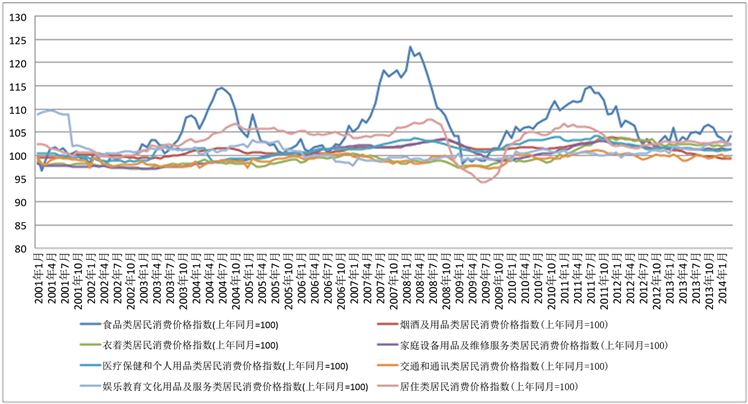Figure 1. Fluctuations of eight types of CPI from 2001.1~2015.2Table 2. Variance of various types of CPI

4. 估计过程

4.1. 模型建立

$\left[\begin{array}{c}\Delta {y}_{t}\\ \Delta {\pi }_{t}\\ \Delta {f}_{t}\\ \Delta {s}_{t}\\ \Delta {l}_{t}\end{array}\right]\left[\begin{array}{ccccc}{\Phi }_{11}\left(L\right)& {\Phi }_{12}\left(L\right)& {\Phi }_{13}\left(L\right)& {\Phi }_{14}\left(L\right)& {\Phi }_{15}\left(L\right)\\ {\Phi }_{21}\left(L\right)& {\Phi }_{22}\left(L\right)& {\Phi }_{23}\left(L\right)& {\Phi }_{24}\left(L\right)& {\Phi }_{25}\left(L\right)\\ {\Phi }_{31}\left(L\right)& {\Phi }_{32}\left(L\right)& {\Phi }_{33}\left(L\right)& {\Phi }_{34}\left(L\right)& {\Phi }_{35}\left(L\right)\\ {\Phi }_{41}\left(L\right)& {\Phi }_{42}\left(L\right)& {\Phi }_{43}\left(L\right)& {\Phi }_{44}\left(L\right)& {\Phi }_{45}\left(L\right)\\ {\Phi }_{51}\left(L\right)& {\Phi }_{52}\left(L\right)& {\Phi }_{53}\left(L\right)& {\Phi }_{54}\left(L\right)& {\Phi }_{55}\left(L\right)\end{array}\right]\left[\begin{array}{c}{v}_{1t}\\ {v}_{2t}\\ {v}_{3t}\\ {v}_{4t}\\ {v}_{5t}\end{array}\right]$ ,

${\Phi }_{11}\left(L\right)=0,\text{\hspace{0.17em}}{\Phi }_{13}\left(L\right)=0,\text{\hspace{0.17em}}{\Phi }_{14}\left(L\right)=0,\text{\hspace{0.17em}}{\Phi }_{15}\left(L\right)=0$ .

${\Phi }_{24}\left(L\right)=0,\text{\hspace{0.17em}}{\Phi }_{25}\left(L\right)=0$ .

${\Phi }_{31}\left(L\right)=0,\text{\hspace{0.17em}}{\Phi }_{34}\left(L\right)=0$ .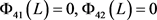.

4.2. 模型估计

4.2.1. 平稳性检验

VAR模型要求系统具有平稳性，因此首先对各个变量进行ADF单位根检验  。取5%的显著性水平，发现原序列除 ${y}_{t}$ 外其他各变量均不平稳。因此对其他变量进行一阶差分再进行检验，最终得到平稳序列。因此最后选定进行模型构建的变量为 ${y}_{t}$$d{\pi }_{t}$$d{f}_{t}$$d{s}_{t}$$d{l}_{t}$

4.2.2. 滞后阶数确定及AR根检验Table 3. Results of ADF Unit Root Test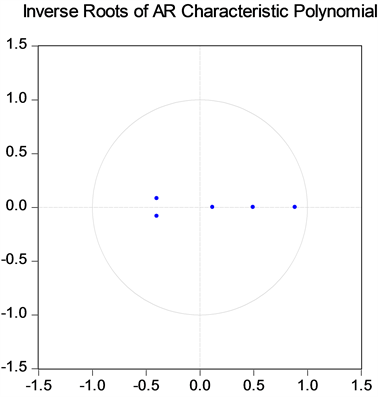Figure 2. Plot of Inverse Roots of AR Characteristic Polynomial

AR根图显示各特征根均在单位圆内，因此VAR稳定，满足脉冲响应所需条件。SVAR模型估计结果见附录1。

4.2.3. 脉冲响应分析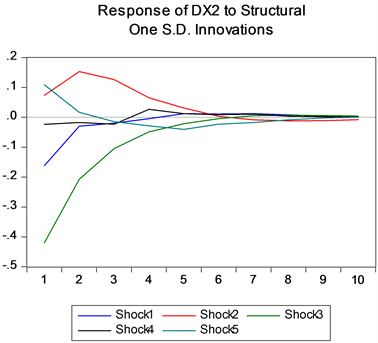Figure 3. Response of CPI to various shocks

4.2.4. 核心CPI估计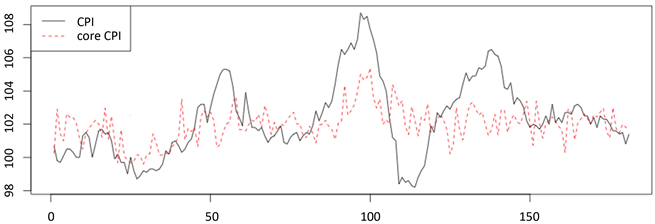Figure 4. Time series plot of CPI v.s. core CPI

5. 讨论

1. SVAR估计结果

2. 脉冲响应图表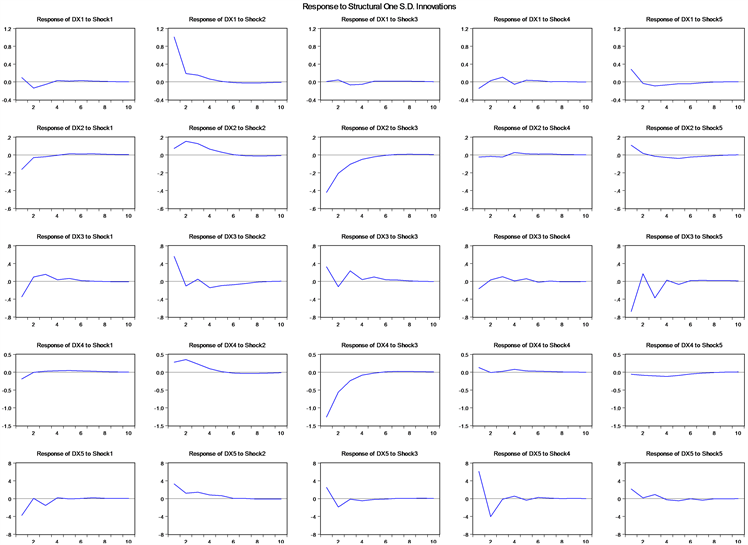3. 核心CPI估计结果

  Rich, R.W. and Steindel, C. (2005) A Review of Core Inflation and an Evaluation of Its Measures. FRB of New York Staff Report No. 236. https://ssrn.com/abstract=873860 https://doi.org/10.2139/ssrn.873860  Bryan, M.F. and Cecchetti, S.G. (1993) Measuring Core Inflation. NBER Working Papers, 49, 279-312. https://doi.org/10.3386/w4303  卢满生. 我国通货膨胀指数修正研究[D]: [博士学位论文]. 成都: 西南财经大学, 2010.  张延群. 中国核心通货膨胀率的度量及其货币政策涵义[J]. 金融研究, 2011(1): 64-72.  赵留彦. 中国核心通胀率与产出缺口经验分析[J]. 经济学(季刊), 2006, 5(3): 1197-1218.  郭朋, 吴烨. 我国核心通胀率的决定因素: 基于面板数据的实证研究[J]. 国际金融研究, 2011(9): 43-50.  张欣. 中国核心通货膨胀的测度与分析[D]: [博士学位论文]. 大连: 东北财经大学, 2013.  巴曙松, 韩明睿. 基于SVAR模型的金融形势指数[J]. 宏观经济研究, 2011(4): 26-31.  李标. 对中国自然失业率的估计—基于SVAR模型的实证分析[D]: [硕士学位论文]. 成都: 西南财经大学, 2011.  陈涤非, 李红玲, 王海慧, 等. 通胀预期形成机理研究—基于SVAR模型的实证分析[J]. 国际金融研究, 2011(3): 29-36.  齐红倩, 黄宝敏. 中国居民消费需求变动原因分解—基于SVAR模型的分析[J]. 数量经济研究, 2012, 3(2): 70-80.  李毅, 周伍阳. 基于SVAR模型的我国通货膨胀动态响应机制研究[J]. 商业经济研究, 2013(29): 73-74.  赵昕东. 基于SVAR模型的中国核心通货膨胀的估计与应用[J]. 统计研究, 2008, 25(7): 45-51.  武晓婷. 我国核心通货膨胀的SVAR模型的分析研究[D]: [硕士学位论文]. 北京: 首都经济贸易大学, 2012.  汤丹, 赵昕东. 中国核心通货膨胀的估计—基于贝叶斯Gibbs Sampler状态空间模型[J]. 数量经济研究, 2011(1): 39-46.  王毅, 石春华, 叶欢. 核心通货膨胀测度与应用[R]. 北京: 中国人民银行, 2015-01.  高铁梅. 计量经济学分析方法与建模EViews应用及实例[M]. 北京: 清华大学出版社, 2009.